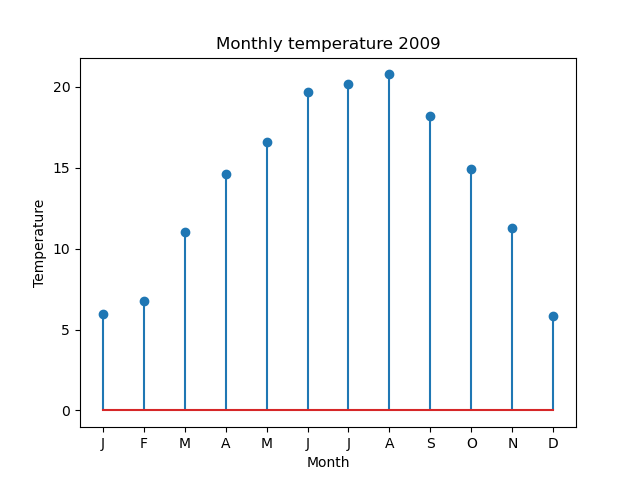# Stem plots in Matplotlib

By Martin McBride, 2022-07-09
Tags: matplotlib stem plot
Categories: matplotlib numpyA stem plot is quite similar to a bar chart. The difference is that, instead of using a bar for each data point, a stem plot uses a vertical line with a marker at the top.

Here is an example stem plot:This is very similar to the bar chart we created earlier.

Stem plots can be useful if there are a lot of data points. Since the line and marker occupy less area than the bar, the plot looks less cluttered. This is largely a matter of personal taste.

The code is very similar:

import matplotlib.pyplot as plt
import csv

with open("2009-temp-monthly.csv") as csv_file:
temperature = [x for x in csv_reader]

month_names = ["J", "F", "M", "A", "M", "J",
"J", "A", "S", "O", "N", "D"]

months = range(12)

plt.title("Monthly temperature 2009")
plt.xlabel("Month")
plt.ylabel("Temperature")
plt.xticks(months, month_names)

plt.stem(months, temperature)
plt.show()


This code is available on github as stemplot-monthly-temperatures.py.

The only difference is the call to plt.stem rather than plt.bar.

## Styling stem plots

We can style a stem plot in a similar way to a line plot or scatter plot, with the following optional parameters:

• The fmt parameter uses a string to specify basic colour, line style, and marker shape options. Use this for simple formatting.
• The color parameter sets the line colour, using named colours of RGB values.
• The linewidth parameter sets the width of the line.
• The linestyle parameter can create dashed lines of various types.
• The marker, markeredgecolor, markeredgewidth, markerfacecolor, and markersize control the marker appearance.

These options are covered in more detail in the article line and marker styles.